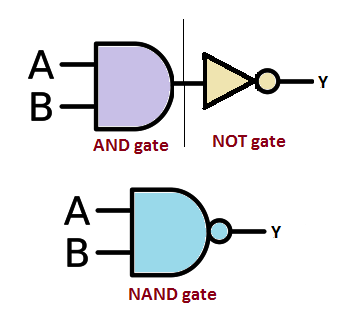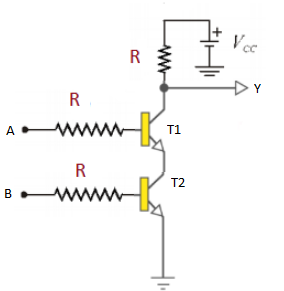# NAND Gate

The digital system is built using many logic gates. The logic gate is an electronic circuit having one or more inputs and a single output. These gates are constructed using diodes and transistors. Boolean algebra is the mathematical expression that explains the output of logic gates.

## Logic gates

The Logic gates are used to carry out logical operations using single or multiple binary inputs and a single binary output. The input and output of a logic gate are based on certain logic, which is explained using Boolean algebra. Boolean algebra uses only two variables zero or one. The most basic type of logic gates are OR gate, AND gate and NOT gate. In addition to the basic logic gate, there are combination gates like NAND gate, NOR gate, XOR gate, etc made by combining basic logic gates in different ways.

## What is a NAND gate?

The NAND gate or “NotAND” gate is the combination of two basic logic gates, the AND gate and the NOT gate connected in series. The NAND gate and NOR gate can be called the universal gates since the combination of these gates can be used to accomplish any of the basic operations. Hence, NAND gate and NOR gate combination can produce an inverter, an OR gate or an AND gate.

The output of a NAND gate is high when either of the inputs is high or if both the inputs are low. In other words, the output is always high and goes low only when both the inputs are high. The logic NAND function is given by the Boolean expression $$Y=\bar{A.B}$$. Here A, B are the inputs and Y is the output.

The Boolean expression given for a NAND gate is that of logical addition and it is opposite to AND gate. The Boolean expression is given by a single dot (.) with an overline over the expression to show the NOT or the logical negation of the NAND gate.

## Symbol and truth table of NAND gate

The symbol of the NAND gate is represented as a combination of AND gate and NOT gate. The Boolean expression is given as $$Y=\bar{A.B}$$.The truth table of a NAND gate is given below

 A B Y 0 0 1 0 1 1 1 0 1 1 1 0

## Transistor NAND gate

A simple NAND gate can be constructed using transistors and resistors as shown in the figure below. The inputs of the NAND gate are directly connected to the transistor bases.Test your Knowledge on Nand Gate